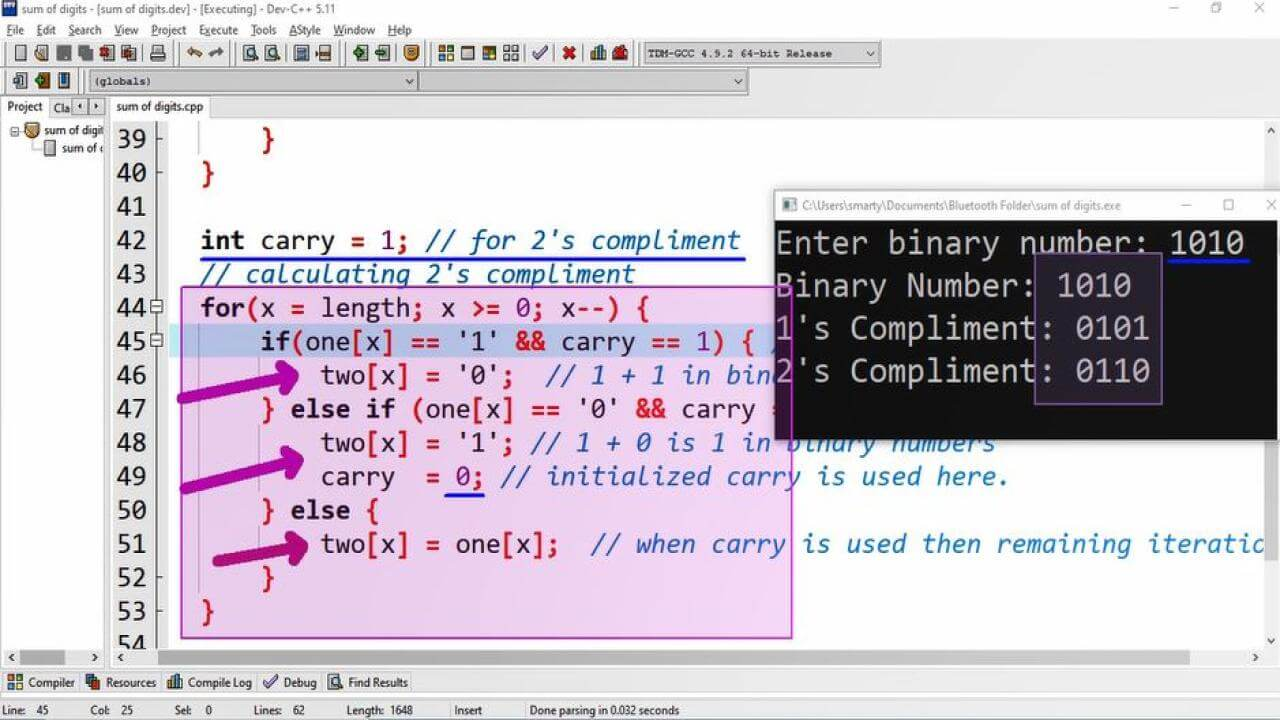# Two Compliment Using For Loop CPP Program

## Source Code:

#include <iostream>

using namespace std;

/*
Logic of 1's and 2's compliment
1's compliment
10101 = 01010
2's compliment
01010
1 +
---------
01011
*/

int main(int argc, char** argv) {

// Declaration statements
int length = 4; // This is defined for binary input
char bin;  // This is used to store binary digit
char one;  // This array will store 1's compliment
char two;  // Used to store 2's compliment
int x;  // used as counter variable
int flag = 0; // used to indicate successful completion

// Taking binary input
cout<<"Enter binary number: ";
gets(bin);  // gets for input in char array

for( x = 0; x < length; x++) { // traversing binaries
if(bin[x] == '1') { // Changing 1 to 0
one[x] = '0';
} else if(bin[x] == '0') { // changing 0 to 1
one[x] = '1';
} else {
cout<<"Invalid Binary Number";
flag = 1;  // indicating unsuccessful conversion
break;  // terminating for loop
}
}

int carry = 1; // for 2's compliment
// calculating 2's compliment
for(x = length; x >= 0; x--) {
if(one[x] == '1' && carry == 1) { // last digit is 1
two[x] = '0';  // 1 + 1 in binary is 0
} else if (one[x] == '0' && carry == 1) { // last digit is 0
two[x] = '1'; // 1 + 0 is 1 in binary numbers
carry  = 0; // initialized carry is used here.
} else {
two[x] = one[x];  // when carry is used then remaining iterations.
}
}

// Checking successful computation
if(flag == 0) {
cout<<"Binary Number: "<<bin<<endl;
cout<<"1's Compliment: "<<one<<endl;
cout<<"2's Compliment: "<<two<<endl;
}
return 0;
}

## Output:## Working:

To calculate two compliment first of all we have to compute one compliment of given binary number. 1's compliment is taken by inverting all binary digits of given binary number. In this program example we declare the following variables for calculation of two compliment.

 Sr. Identifier Data Type Description 1 length int This variable is used to define maximum length of input binary number. 2 bin int array bin variable is used to take binary number input. 3 one int (array) one array variable will store the calculated one's compliment of given binary number. 4 two int (array) This array will store two's compliment from calculated one'1 compliment. 5 flag int flag variable is used to check valid or invalid binary number. 6 x int x is counter variable 7 carry int carry variable is used for 2's compliment

The algorithm first of all calculate the one's compliment using for loop. Here we have declare x variable as counter variable. That will be used in both for loop for 1's and 2's compliment.

To calculate 1's compliment we just traverse the entire binary number array and invert each binary digit. Such as if we found binary digit 0 in bin array we will store 1 in one array and vice versa. This process is simple and easy to understand.

In second phase /  code block we have to calculate two's compliment. as discussed in starting of program 2's compliment is calculated by adding 1 binary digit into calculated 1' compliment. This digit is also called carry bit/digit. So that we declare an additional variable named as carry and initialize it with 1.

In for loop we check if provided binary from MSB (most significant bit) is 0 the 0+1 (carry) will be 1. And there our carry will be consumed and we will set carry as 0. On the other hand if the MSB is 1 then 1+1 is 0 (with 1 carry) in binary numbers so that our carry will remain in process.

When this carry will be used then we just copy the binary digits of one array to two array. After completion this entire process we will print binary number, one's compliment and two's compliment using cout statement.